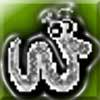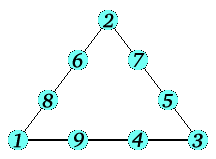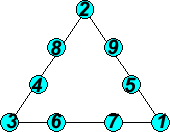#### You may also like### Prompt Cards

These two group activities use mathematical reasoning - one is numerical, one geometric.### Exploring Wild & Wonderful Number Patterns

EWWNP means Exploring Wild and Wonderful Number Patterns Created by Yourself! Investigate what happens if we create number patterns using some simple rules.### Worms

Place this "worm" on the 100 square and find the total of the four squares it covers. Keeping its head in the same place, what other totals can you make?

# Magic Triangle

##### Age 7 to 11 Challenge Level:

Michael and Robert from Necton Middle School in Norfolk sent the following solution, where the sides all add up to 17:Simone from Stroud Green Primary School sent us another solution where the sides all add up to 17.In fact, there are lots more solutions. Here's a list of them (including the two shown above). Each group of four numbers makes up a side of the triangle. Of course, within a side you can switch round numbers that aren't on a corner, but that doesn't really give any new solutions.

17 = 1 + 5 + 9 + 2 = 2 + 4+ 8+ 3 = 3 + 6 + 7 + 1
17 = 1 + 6 + 8 + 2 = 2 + 5 + 7 + 3 = 3 + 4 + 9 + 1

19 = 1 + 5 + 9 + 4 = 4 + 2 + 6 + 7 = 7 + 3 + 8 + 1
19 = 1 + 6 + 8 + 4 = 4 + 3 + 5 + 7 = 7 + 2 + 9 + 1
19 = 2 + 5 + 9 + 3 = 3 + 1 + 8 + 7 = 7 + 4 + 6 + 2
19 = 2 + 6 + 8 + 3 = 3 + 4 + 5 + 7 = 7 + 1 + 9 + 2

20 = 1 + 6 + 8 + 5 = 5 + 2 + 4 + 9 = 9 + 3 + 7 + 1
20 = 2 + 4 + 9 + 5 = 5 + 1 + 6 + 8 = 8 + 3 + 7 + 2
20 = 2 + 6 + 7 + 5 = 5 + 3 + 4 + 8 = 8 + 1 + 9 + 2
20 = 3 + 4 + 8 + 5 = 5 + 2 + 6 + 7 = 7 + 1 + 9 + 3
20 = 4 + 2 + 9 + 5 = 5 + 1 + 8 + 6 = 6 + 3 + 7 + 4
20 = 4 + 3 + 8 + 5 = 5 + 2 + 7 + 6 = 6 + 1 + 9 + 4

21 = 3 + 5 + 7 + 6 = 6 + 2 + 4 + 9 = 9 + 1 + 8 + 3
21 = 3 + 4 + 8 + 6 = 6 + 1 + 5 + 9 = 9 + 2 + 7 + 3
21 = 3 + 2 + 9 + 7 = 7 + 1 + 5 + 8 = 8 + 4 + 6 + 3
21 = 3 + 5 + 6 + 7 = 7 + 2 + 4 + 8 = 8 + 1 + 9 + 3

23 = 7 + 2 + 6 + 8 = 8 + 1 + 5 + 9 = 9 + 3 + 4 + 7
23 = 7 + 3 + 5 + 8 = 8 + 2 + 4 + 9 = 9 + 1 + 6 + 7